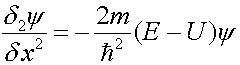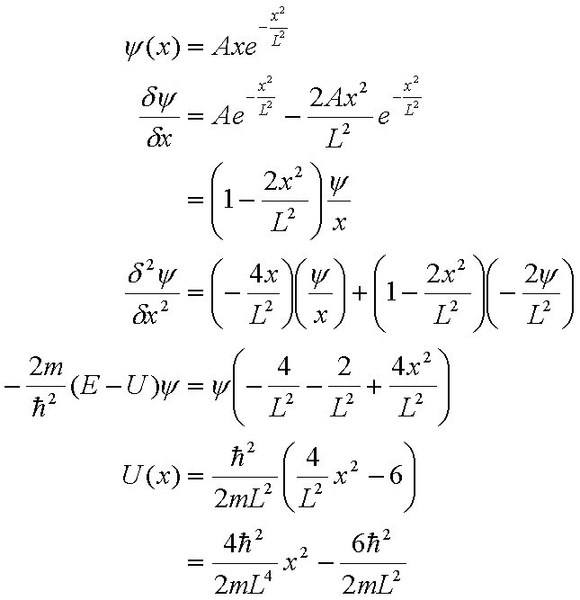# Schrodinger's equation and potential energy

xiankai

## Homework Statement

1. In a region of space, a particle with zero total energy E has a wavefunction

ψ (x) = A x exp - (x2/L2)

a) Find the potential energy U as a function of x
b) Make a sketch of U(x) versus x

## Homework Equations

time independent schrodinger's equation## The Attempt at a Solutionjudging by the nature of the equation, the graph of U(x) against x seems to be that of a quadratic curve with the minimum point below the y-axis.

did i get it correct? im abit uncertain...